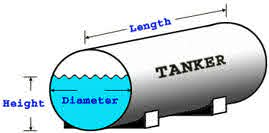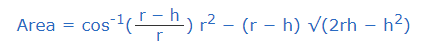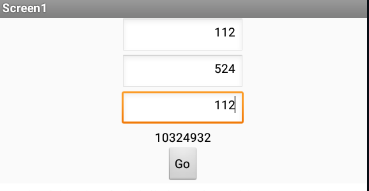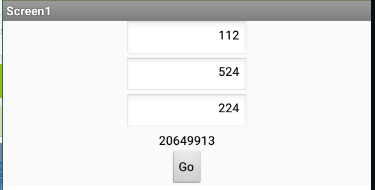# How to use math formula given

I am new in mit app inventor
Plz help me
How use this exel formula in my app

Hear D16 is value

=(((112^2*(((D16-112)/112)mg*SQRT(1-((D16-112)/112)^2)+ASIN((D16-112)/112)+PI()/2))*524)*0.001)/1000

use the math blocks from the math drawer

It would really help if you provided a screenshot of your relevant blocks, so we can see what you are trying to do, and where the problem may be.

To get an image of your blocks, right click in the Blocks Editor and select "Download Blocks as Image". You might want to use an image editor to crop etc. if required. Then post it here in the community.

Taifun

Trying to push the limits! Snippets, Tutorials and Extensions from Pura Vida Apps byTaifun.

I see from your formula you are using the ASIN() excel function.

Be aware that Excel measures angles in radians, not degrees, so see this library for conversion help ...

Also, your block structure will be easier to check if you switch to external inputs (right click on the block.)

(sample video)

I reformatted your blocks to show the nesting more easily, so we can check the math:
(draggable)

But it is impossible to make sense of this without a diagram of tank 12 and its geometry,
particularly where the magic number 112 comes from.

1 Like

there 112 is radius of tank , 524 is length of tank and oping dip or closing dip is Dip of tank measure by dip tape in centimeter
at the last in equation multiplay by 0.001 is use for convert volume to liter
and divided by 1000 to convert in kilolitre

i am use given below formula in exel to mesure how many litre product in the horizantol
tank
=(((112^2*(((D16-112)/112) *SQRT(1-((D16-112)/112)^2)+ASIN((D16-112)/112)+PI()/2))*524)*0.001)/1000

here D16 is dip of tankSo the dip of the tank is the length of the wet portion of a dip stick that has been lowered all the way down into the tank from the top, i.e. if the dip = the diameter the tank is full?

Also, what is the meaning of "mg" in your formula?

Sorry mg is my typing mistake

DIP is hight of product in the tank

524 is length of tank

Okay, thanks, we have enough now to proceed to the blocks.

I ran into some trouble crossing the equator of the tank, and had to improvise. I couldn't find any formula using arcsin, so I used arccosine formulas, which were accompanied by good explanations.
https://www.mathsisfun.com/geometry/cylinder-horizontal-volume.html
works up until the equator (yielding negative area over the equator), so I flipped the problem (water<==>air) for height > radius,
coming up with a recursive formula.

I skipped the part where you convert from cubic centimeters (cm) to kilos or whatever.
Do that OUTSIDE my formulas, to keep our sanities.tanks_a_lot.aia (43.8 KB)Regarding your multiple tanks, keep your tank sizes in csv tables from a file, loaded at app start, and pass the R and L to the procedure. Never open up the procedure for further customization, just wrap the calls.